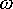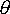QUESTION #256

# What is the angular velocity of the Earth around the Sun? How do we get it?

We know the Earth goes round the Sun, all the way around is 2radians (360 degrees). We also know that it takes a year (approx 365 days) which is therefore about 3.2x107 secs.
Therefore= 2/ 3.2x107 = 2.0x10-7 rad/s. We have calculated the angular velocity.
However, if we can measure the distance to the Sun we can also calculate the velocity of the Earth relative to the Sun. Although unless we define a direction this is more technically known as the speed. This can be done by looking at the definition of the radian. The radian is a unit which conects the radius of an arc, the length of the arc and the angle subtended by the arc. The formula for this is s = r x(where s is the length of the arc, r is the radius andthe angle). So if we know the radius of the Earth's orbit (1.5x1011m) we can substitute the angular velocity from our previous equation to give v =x r (where v is the velocity,the angular velocity and r the radius).